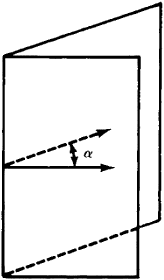# Dihedral Angle

(redirected from Dihedral angles)
Also found in: Dictionary.

## dihedral angle

[dī′hē·drəl ‚aŋ·gəl]
(mathematics)
The angle between two planes; it is said to be zero if the planes are parallel; if the planes intersect, it is the plane angle between two lines, one in each of the planes, which pass through a point on the line of intersection of the two planes and are perpendicular to it.

## Dihedral Angle

a figure formed in space by two half-planes emanating from a single straight line and also the portion of space bounded by these half-planes (see Figure 1).Figure 1.

The half-planes are called the faces of the dihedral angle and their common straight line the edge. The dihedral angle is measured by the linear angle, that is, the angle α between two perpendiculars to the edge that emanate from one point and that lie in the different faces or, in other words, the angle formed by the intersection of the dihedral angle and a plane perpendicular to the edge.

References in periodicals archive ?
We now show that all dihedral angles are submutliples of [pi].
An ideal polytope P is called Coxeter if the dihedral angles of edges of P are submultiples of [pi].
SAR studies of the ten synthetic molecules demonstrated that the three-dimensional shape of the t-Bu attached side chain significantly affects the potent neurotoxicity of 1: the bulkiness of the C9-substituent and the specific dihedral angle of the C7-C8 bond strongly correlated to potent cytotoxicity, and truncation of the terminus induced activity loss.
The decrease in the dihedral angles between the two adjacent subunits induced by the presence of [pi]-spacers groups is in the order of PFEBT > PFVBT > PFDTBT > PFBT, which increases the effective conjugation degree.
Dihedral angle checks Ramachandran plot shows phi-psi distribution.
30) The visible angle made by two curved lines may be equated with the dihedral angle of the planes containing them.
The difficulty is caused by overlap of van der Waals radii and repulsion before the two ends of a chain may become joined and, in the ring, by repulsions between transannular hydrogens and by unfavorable dihedral angles.
The panels of a geodesic dome can be TABULAR DATA OMITTED assembled without any attempt to maintain specific dihedral angles.
Aconvexpolyhedron P of finite volume in the n-dimensional hyperbolic space Hn is called a Coxeter polyhedron if its dihedral angles are submultiples of [pi].

Site: Follow: Share:
Open / Close